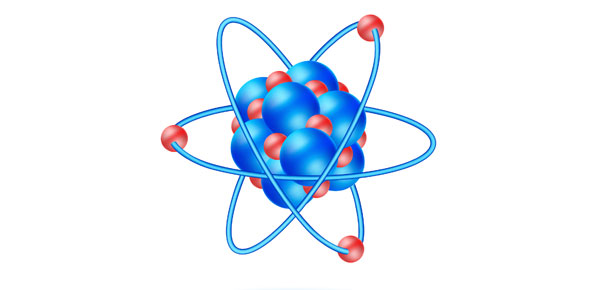# Physical Science

5 Questions | Total Attempts: 121To see if we got science questions down and if we understand it or not. But if you know you science than congrats if you don't continue studying.

Related Topics
• 1.
When is an atom unlikely to react ?
• A.

An atom is unlikely to react because when the highest occupied energy level of an atom is filled w| electrons, the atom is stable.

• B.

An atom is unlikely to react when because when the highest occupied energy level of an atom is filled w| protons and the atom is unstable

• C.

An atom is unlikely to react because when the lowest occupied energy level of an atom is filled with protons, the atom is stable

• D.

An atom is unlikely to react because when the highest occupied energy level of an atom is filled with protons, the atom is unstable

• 2.
What does an electron dot diagram shows and why is it helpful ?
• A.

An electron dot diagram is a is a model of the nucleus in which each dot represents a v.e [valence electron]. Its helpful because is shows how many protons are represented & how likely an atom is to react .

• B.

An electron dot diagram is a model of an atom in which each dot represents a proton . Its helpful because it shows how many v.e [ valence electron ] are present & how likely an atom is to react .

• C.

An electron dot diagram is a model of an atom in which each dot represents a v.e [ valence electron ] Its helpful because it shows how many v.e are present & how likely an atom is to react .

• D.

An electron dot diagram is a model of the nucleus in which each dot represents a proton . Its helpful because it shows how many protons are present & how likely an atom is to react .

• 3.
What is one way in which atoms of elements can achieve stable electron configuration ?
• A.

One way in which atoms of elements can achieve unstable electron configuration is through the transfer of the v.e [ valence electron ] between the nucleus

• B.

One way in which atoms of elements can achieve stable protons configuration is through the transfer of v.e [ valence electron ] between the atom

• C.

One way in which of elements can achieve stable electrons configuration is through the transfer of protons between atom.

• D.

One way in which atoms of elements can achieve stable electrons configuration is through the transfer of v.e [ valence electron ] between atom .

• 4.
Describe the process of ionic bounding ?
• A.

Ionic Bounding occurs when an atom gives its v.e to another atom which gains the electron .

• B.

Ionic bounding occurs when an element give its w.e to another element which gains the electron.

• C.

Ionic bounding occurs when an atom gives protons to another element which gains the electron

• D.

Ionic bounding occurs when an atom gives its v.e to another element in which gains an the proton

• 5.
How does ionization energy affects the process ?
• A.

The amount of energy used to remove an electron is called ionization energy & electrons need to be removed in order to be transfered .

• B.

The amount of energy used to remove a proton is called ionization energy & protons need to be removed in order to be transfered .

• C.

The amount of energy used to remove a proton is called ionization energy & electrons needs to e removed in order to be transfered .

• D.

The amount of energy used to be remove a electron is called ionization energy & protons need to be removed in order to be transfered.